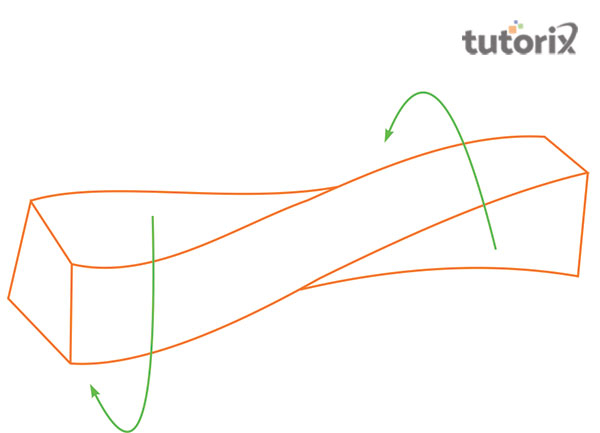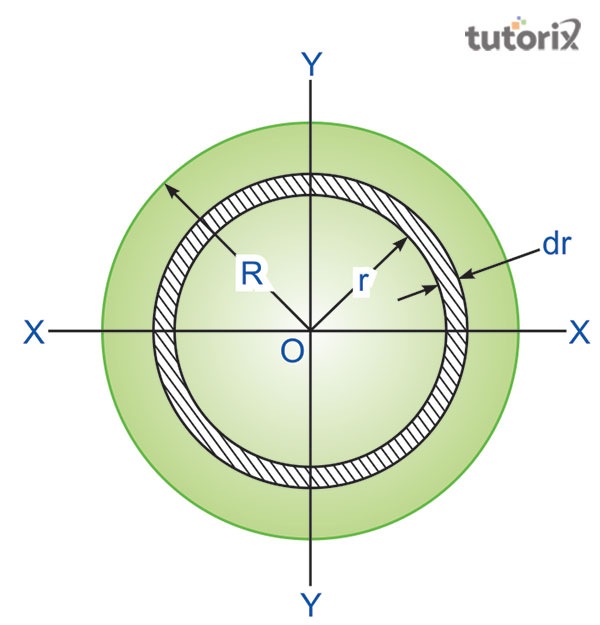# Derivation of Torsion Equation

## Introduction

In the field of solid mechanics, it can be found that twisting a material by applying a torque on it is denoted as a torsion equation or torsion constant. In various ways, torsion can be expressed. Those possible ways are pascals (Pa), Newton-meters (Nm), or even in foot pound-force (ft. lbf). The SI unit of torsion constant is Newton per square meter (N/m2) or pounds per square inch (p/inc2).

There the process of wrapping is called distortion occurring simultaneously with the occurrence of twisting in non-circular sections. The traverse becomes harsh while the process of wrapping. The equation of torsion is also referred to as the torsion constant. In the case of the derivation of the pure torsion equation, it can be stated that Hooke’s law must be followed along with the content.

It is vital for the circular segment to remain circular. Here the stress of the particles never exceeds its limit of elasticity. The cross-sectional area of the cross-sectional region must remain plane.

## What is torsion?Figure 1: Torsion

In solid mechanics, the torsion is associated with the feature of twisting a particular body because of the application of torque to it. Torsion can further be described in either the scale of Pascal (Pa) or in terms of the SI unit that is Newton per square meter it is also mentioned in terms of pounds per square inch or psi.

However, in contrast to this, the torque is denoted in Newton-meters (Nm) or the foot pound-force. Certain sections in a given object act perpendicular to the acting torque axis. In these particular sites the resultant shear stress is perpendicular to the radius. In a given cross-section that is not circular in shape, a distortion occurs along with the twisting; this particular distortion is termed warping. At the site of warping the transverse section are coarse.

## Torsion constant

The torsion constant is associated with the geometrical property found in the crosssection of a bar, this property exists related to the applied torque through the bar of the axis and the angled formed by the twist. All these particular theories are associated with a homogenous plastic bar. As opined by Atavin et al. (2018), the torsional stiffness of a bar is governed by the torsion constant that is combined with different properties of the object like the length. The SI unit of Torsion constant is denoted by m.

## Torsion equation derivationFigure 2: Torsion equation for circular shaft

A solid circular shaft is considered with a designated radius R and it is associated with the torque T that acts on both the ends under the same amount of torque (hkdivedi, 2022).

$\mathrm{Angle\: of\: radius=\frac{arc}{radius}}$

$\mathrm{Arc\: AB = R\theta = L\gamma }$

$\mathrm{\gamma =\frac{R\theta }{L}}$

Here, the two distinct fixed points associated with the circular shaft are A and B

And, γ is the angled formed by AB

The modulus of rigidity is denoted by $\mathrm{G=\frac{\tau }{\gamma } }$

Here, the shear stress is denoted by𝜏 and the shear strain is denoted by γ

$\mathrm{\frac{\tau }{G}=\Gamma }$

There it can be established that $\mathrm{\frac{R\theta }{L}=\frac{\tau }{G} }$

A small strip of radius with a thickness dr is considered that undergoes shear stress.

$\mathrm{\Gamma'*2\pi rdr}$

Here, r is the radius of a small strip

The thickness of the strip is denoted by dr

The sheer stress is γ

The torque at the centre of the shaft $\mathrm{2\pi\, \Gamma'\, r^{2}dr}$

$\mathrm{T=\int_{0}^{R}2\pi\, \Gamma'\, r^{2}dr}$

$\mathrm{T=\int_{0}^{R}2\pi\, \frac{G\theta }{L}\, r^{3}dr}$

$\mathrm{T=\frac{2\pi\,G\theta }{L}\int_{0}^{R}r^{3}dr=\frac{G\theta }{L}\left [ \frac{\pi d^{4}}{32} \right ]}$

Substituting and integrating the value of R

$\mathrm{\frac{G\theta }{L}J}$

Therefore, substitution for polar moment of inertia leads to

$\mathrm{\frac{T }{J}=\frac{\tau }{r}=\frac{G\theta }{L}}$

## Assumption of torsion equation

• The material displays the property of elasticity (Engineeringtoolbox, 2022).

• The object is needed to abide by the Hooke’s law

• The shear stress is out to be proportional to the shear strain

• The area of the cross-section is needed to be plane.

• The circular section is needed to be circular

• The diameter of the material is needed to rotate through a particular angle

• The stress existing in the material should never be higher than the elastic limit

These are assumptions that are needed to be followed by the material to obtain desired results.

## Conclusion

The torsion equation mainly follows the rule of Hooke’s law through the entire content of its derivation. The materials that are incorporated here are uniform in nature means they have elastic properties. One of the main assumptions that can be followed to derive a proper torsion equation, is that shear stress must remain proportional to shear strain in the particle. Some sections here remain perpendicular to the axis of torque. The sheer stress resultant also remains perpendicular to the radius. In the context of the shear stress in the shaft, it can be mentioned that if the shaft is subject to torque and twisting then the shaft the shearing stress will be formed.

## FAQs

Q1. What do you mean by Torsion constant?

Ans. Torsion constant is denoted as the geometrical property of cross-sectional bars that remains involved in the bar axis. In this situation, there is a connection between applied torque and twisting angle that has the SI unit: m4.

Q2. What is the concept of torque?

Ans. In mechanics, torque is denoted as the force that occurs in rotational movement or rotational motion. The torque is also determined as rotational force, a moment of force, and the turning effect.

Q3. What are the derivation assumptions of the Torsion equation?

Ans. The assumption of the derivation of the torsion equation includes it follows Hooke’s law throughout the entire content of the derivation of the equation. The material used in torsion must have shear stress proportional to shear strain. The circular partition must remain circular and materials’ stress must not exceed the elasticity limit.

Q4. What do you mean by Torsion?

Ans. The twisting and wrenching of a body with the help of exertion of any particular force is regarded as torsion. The state of being twisted and the nature of twisting are the two most important parts of torsion.

Updated on: 13-Oct-2022

3K+ Views